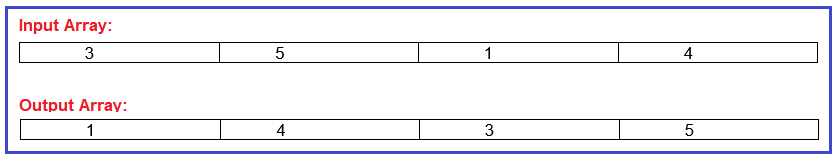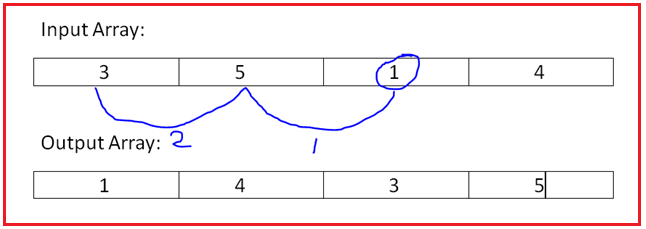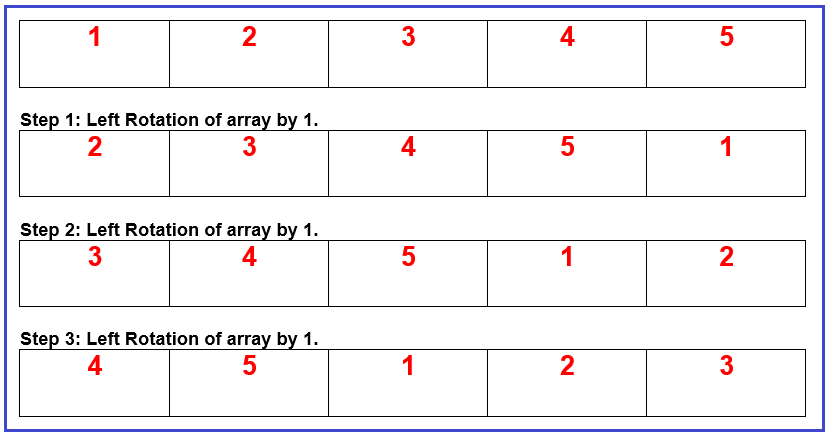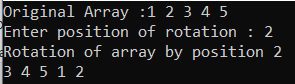# Rotate an array by K position using Popup and Unshifting Algorithm in C#

## Rotate an array by K position using Popup and Unshifting Algorithm in C#

In this article, I am going to discuss Rotate an array by K position using Popup and Unshifting Algorithm in C# with Examples. Please read our previous article where we discussed Right Rotation of Array by 1 in C#. Rotation of an array basically means shifting each and every element to specified positions to the left or right. While writing the program for array rotation we should consider 2 factors:

1. The direction of Rotation – In what direction do we want to perform the rotation. Whether it would be left to right (clockwise) or right to left (anticlockwise).
2. Position of Rotation – At which position rotation will start.

Note: Rotation of array by position k (1,2,3 etc. depends on user input) means we need to shift the elements to the left or right side by k position.

##### Example: Rotate this array by position 2So, by position 2 means each element will be shifted to the left side 2 times like 1 has shifted from index 2 to index 0 (2-0 = 2) as shown in the below fig.Popup and Unshifting Algorithm is same as Rotation of array by 1 which we have discussed earlier. Let’s understand this with an example, suppose we want “3” as the output on our screen we can get this by adding 1 to 3 times i.e., (1+1+1=3). Same way, if we want to rotate an array by the position of 3 we can do this by doing a rotation of the array by 1 to 3 times.

Which is,
1st Time: Rotation of array by 1,
2nd Time: Rotation of array by 1,
3rd Time: Rotation of array by 1
And we will get our Rotation of array by 3.

##### Demonstration:So, as you can see from the above fig. We have successfully completed the rotation of the array of position 3. The element 4 at index 3 has been shifted to index 0 (3-0). Likewise, all the elements have been shifted to position 3.

##### Algorithm to rotate an array by 1 (Left Rotation)

Step1: Create a Temp variable to store the value at index 0.
Step2: Sift all the elements of array to left side by position 1 i.e. arr[i] = arr[i+1];
Step3: Store the value of temp at the last index of array i.e. arr[(arr.Length – 1)] = x;

##### Algorithm to rotate an array by k

Step1: Take the value of position from the user and initialize a variable k with 0.
Step3: Call the function of left rotation of array by 1.
Step4: Increment the value of k i.e. k++;
Step5: Repeat steps 3 & 4 till the k value is less than the position value.
Step6: Display the rotated array.

##### Example:

The following C# Program performs rotation of array by position k using popup and unshifting algorithm.

```using System;
namespace DotNetTutorials
{
public class RotationOfArray
{
static void Main(string[] args)
{
int[] arr = new int[] { 1, 2, 3, 4, 5 };
int position = 0, k = 0;
Console.Write("Original Array :");
for (int i = 0; i < arr.Length; i++)
{
Console.Write(arr[i] + " ");
}
Console.WriteLine();

Console.Write("Enter position of rotation : ");

RotationOfArray obj = new RotationOfArray();
while (k < position)
{
obj.LeftRotate(arr);
k++;
}

Console.WriteLine("Rotation of array by position " + position);
for (int i = 0; i < arr.Length; i++)
{
Console.Write(arr[i] + " ");
}

}

void LeftRotate(int[] arr)
{
int x = arr;
for (int i = 0; i < (arr.Length - 1); i++)
{
arr[i] = arr[i + 1];
}
arr[(arr.Length - 1)] = x;
}
}
}
```
###### Output:In the next article, I am going to discuss Rotate an array by K position using reversal algorithm in C# with Examples. Here, in this article, I try to explain Rotate an array by K position using Popup and Unshifting Algorithm in C# with Examples and I hope you enjoy this Rotate an array by position k by using Popup and Unshifting Algorithm in C# article.